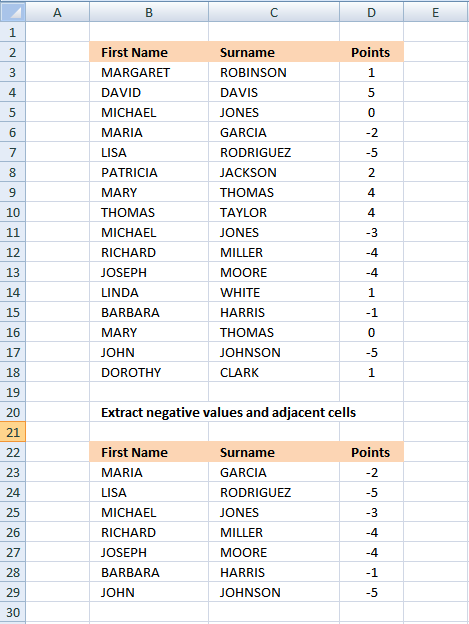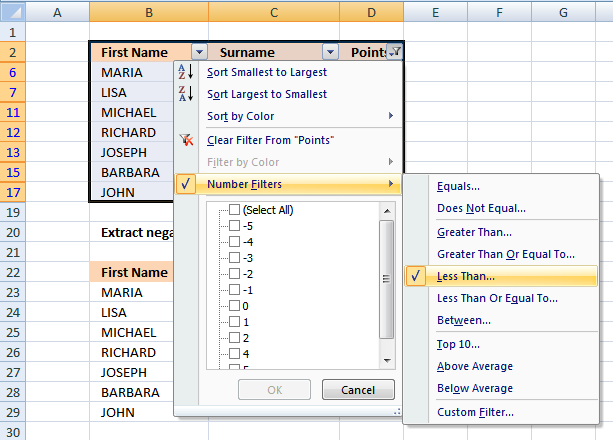Author: Oscar Cronquist Article last updated on February 20, 2019

Extract negative values and adjacent cells (array formula)
Extract negative values and adjacent cells (Excel Filter)Array formula in B23:

=INDEX(\$B\$3:\$D\$18, SMALL(IF(\$D\$3:\$D\$18<0, ROW(\$D\$3:\$D\$18)-MIN(ROW(\$D\$3:\$D\$18))+1, ""), ROW(A1)), COLUMN(A1)) + CTRL + SHIFT + ENTER

copied down as far as needed and then copied to the right as far as needed.

### Explaining array formula in cell B23

Step 1 - Filter negative values and return their corresponding row number

IF(\$D\$3:\$D\$18<0, ROW(\$D\$3:\$D\$18)-MIN(ROW(\$D\$3:\$D\$18))+1, "")

becomes

IF({1;5;0;-2;-5;2;4;4;-3;-4;-4;1;-1;0;-5;1}<0, ROW(\$D\$3:\$D\$18)-MIN(ROW(\$D\$3:\$D\$18))+1, "")

becomes

IF({FALSE; FALSE; FALSE; TRUE; TRUE; FALSE; FALSE; FALSE; TRUE; TRUE; TRUE; FALSE; TRUE; FALSE; TRUE; FALSE}, ROW(\$D\$3:\$D\$18)-MIN(ROW(\$D\$3:\$D\$18))+1, "")

becomes

IF({FALSE; FALSE; FALSE; TRUE; TRUE; FALSE; FALSE; FALSE; TRUE; TRUE; TRUE; FALSE; TRUE; FALSE; TRUE; FALSE}, {1; 2; 3; 4; 5; 6; 7; 8; 9; 10; 11; 12; 13; 14; 15; 16}, "")

and returns

{"";"";"";4;5;"";"";"";9;10;11;"";13;"";15;""}

Step 2 - Return the k-th smallest row number

SMALL(IF(\$D\$3:\$D\$18<0, ROW(\$D\$3:\$D\$18)-MIN(ROW(\$D\$3:\$D\$18))+1, ""), ROW(A1))

becomes

SMALL({"";"";"";4;5;"";"";"";9;10;11;"";13;"";15;""}, ROW(A1))

becomes

SMALL({"";"";"";4;5;"";"";"";9;10;11;"";13;"";15;""}, 1)

and returns 4.

Step 3 - Return value from cell range

INDEX(\$B\$3:\$D\$18, SMALL(IF(\$D\$3:\$D\$18<0, ROW(\$D\$3:\$D\$18)-MIN(ROW(\$D\$3:\$D\$18))+1, ""), ROW(A1)), COLUMN(A1))

becomes

INDEX(\$B\$3:\$D\$18, 4, 1)

becomes

INDEX({"MARGARET","ROBINSON",1; "DAVID","DAVIS",5; "MICHAEL","JONES",0; "MARIA","GARCIA",-2; "LISA","RODRIGUEZ",-5; "PATRICIA","JACKSON",2; "MARY","THOMAS",4; "THOMAS","TAYLOR",4; "MICHAEL","JONES",-3; "RICHARD","MILLER",-4; "JOSEPH","MOORE",-4; "LINDA","WHITE",1; "BARBARA","HARRIS",-1; "MARY","THOMAS",0; "JOHN","JOHNSON",-5; "DOROTHY","CLARK",1} 4, 1)

and returns "Maria" in cell B23.

### Get excel sample file for this tutorial.

extract negative values and adjacent cells.xls
(Excel 97-2003 Workbook *.xls)

### Extract negative values and adjacent cells (Excel Filter)

1. Select B2:D18
2. Press with left mouse button on "Data" tab
3. Press with left mouse button on "Filter" on the Ribbon
4. Press with left mouse button on Black triangle in cell D25. Select "Number Filters"
6. Press with left mouse button on "Less Than..."7. Type 0 (zero)
8. Press with left mouse button on OK!MATCH(lookup_value;lookup_array, [match_type])
Returns the relative position of an item in an array that matches a specified value

IF(logical_test,[value_if:true],[value_if_false])
Checks whether a condition is met, and returns one value if TRUE, and another value if FALSE

SMALL(array,k) returns the k-th smallest row number in this data set.

INDEX(array,row_num,[column_num])
Returns a value or reference of the cell at the intersection of a particular row and column, in a given range

ROW(reference) returns the rownumber of a reference

COLUMN(reference)
returns the column number of a reference